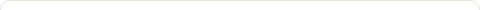# The Euclidean Algorithm

Posted on: October 27, 2010

I recently did a review of an article involving Gaussian integers (complex numbers whose real and “imaginary” parts are integers) in which the Euclidean algorithm was used to find the greatest common divisor (gcd) of two complex numbers. I had never heard of the Euclidean algorithm before, and the process described in the article involved complex numbers and several Greek letters, so I had no idea what was going on. Then, as luck would have it, only a few days later we discussed in math class how to use the Euclidean algorithm to find the gcd of two integers, that is, the largest number that divides both of them without leaving a remainder. It is based on the principle that the gcd does not change if the smaller number is subtracted from the larger number. If this process is repeated, the numbers keep getting smaller until one of them is zero. At this point, the gcd is the larger, non-zero number.

Here is a simple video that describes the process used to find the gcd of 123 and 36:

So what happens if the numbers are a bit larger?

Let’s find the gcd of 5,624 and 3,959:\begin{aligned} 5,624 &= 3,959 \cdot 1 - 1,665\\ 3,959 &= 1,665 \cdot 2 - 629\\ 1,665 &= 629 \cdot 2 - 407\\ 629 &= 407 \cdot 1 - 222\\ 407 &= 222 \cdot 1 - 185\\ 222 &= 185 \cdot 1 - 37\\ 185 &= 37 \cdot 5 - 0 \end{aligned}

So the gcd(5,624, 3,959) = 37.

No matter how large the numbers, the process can be completed in a finite number of steps. It has been found that the maximum number of steps required is five times the number of digits in the smaller integer. If the numbers are sufficiently large, this still could take a very long time. The beauty of this algorithm is that a computer can be programmed to complete the steps in a matter of seconds, rather than the untold hours if calculated by hand. Although I still don’t fully understand this algorithm as applied to Gaussian integers, I have found it to be a wonderful tool for calculations on real integers.

### 2 Responses to "The Euclidean Algorithm"It turns out that the Gaussian integers also have the property that you can discuss divisors (and greatest common divisors in some sense).

The only difference and difficulty is mastering “long division” using Gaussian Integers. This is actually not much different from long division of polynomials (which you are probably familiar with from some time in your math career). Here is a short worksheet with some interesting examples of the Euclidean algorithm using Gaussian Integers, in case you are interested.The Euclidean algorithm should me the mainstream way we teach students how to find the GCF. Why isn’t it? A mystery.